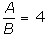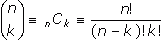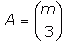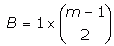Question from Hina (secondary school student):

If one-quarter of all 3-subsets of the integers 1,2,3....,m contain the integer 5, determine the value of m.

How do i go about solving this question?

Hi Hina.

There are two different combinations, one with a 5 and one with no restrictions. Let's call the number of combinations with a five B and the number of combinations with no restrictions A. Thenor simplyNow let's think about the combinations themselves. There are a couple of different notations for choosing k objects from a set of n, but in the end they mean the same thing:In this question, the value A is 3 numbers chosen from a group of m, soFor the other value, B, you first choose the 5 explicitly, so there is just one (1) choice for that. Then you have removed the 5 from the larger set so there are m-1 possible numbers left. You now choose 2 of these, soNow you should have all the pieces of the puzzle to solve the problem. Replace the expressions for A and B in the ratio from earlier and solve for m to get your answer.

Hope this helps,
Stephen and Claude